• The most common use comparison test between two subset of data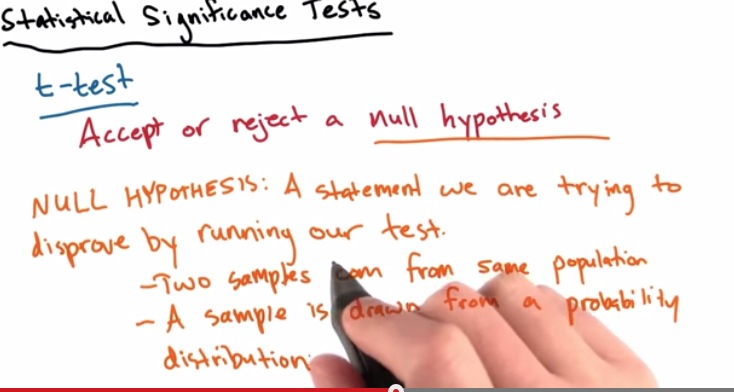• For Example try to make hypothesis that left-hand and right-hand have no different batting average
• t-test is used to approve or decline our null hypothesis, while t-test is parametric test in our statisitic significance test.
• NULL Hypothesis, is hypothesis for our data to be asked, if both of our data is similar? or the sample acquired through data that drawn from normal distribution(same population).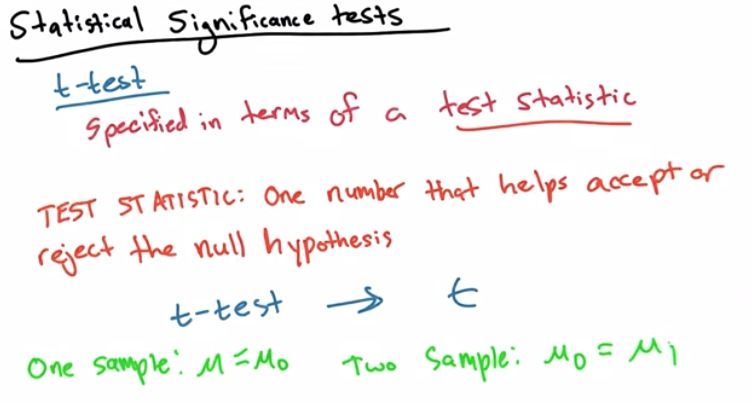• By doing t-test we reduce all the comparison test to one number only, the t value
• if only contain 1 sample, compare average, to average sample 0
• if two samples, compare average 0 to average 1.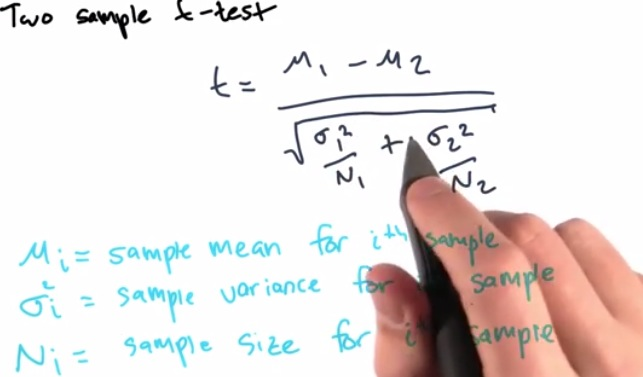• t-value denotes how extreme our results, and probabilty it will reject out null hypothesis

• the v value denotes how many variable took into t calculations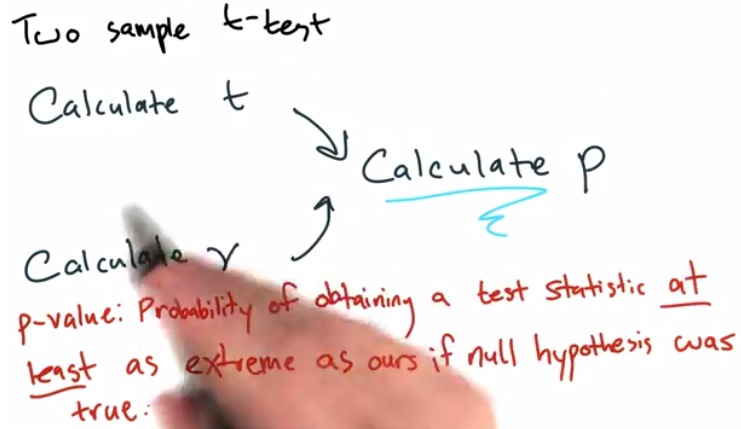• the p value produced by t and v, will then the value that will determine our hypothesis accepted based in our null hypothesis was true
• suppose we want to know the difference batting avg left and right, giveb our null hypothesis both no different
• then if pvalue 0.5, the variance between t and v at least 5% at extreme.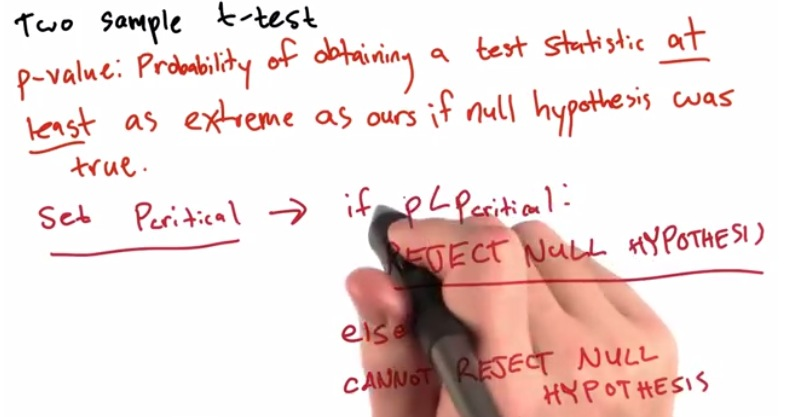• pcritical acts like some kind of threshold value. and it will determine whether our null hypothesis is accepted
• this threshold value will be quite tedious if we set it manually. rather, it can be done automatically.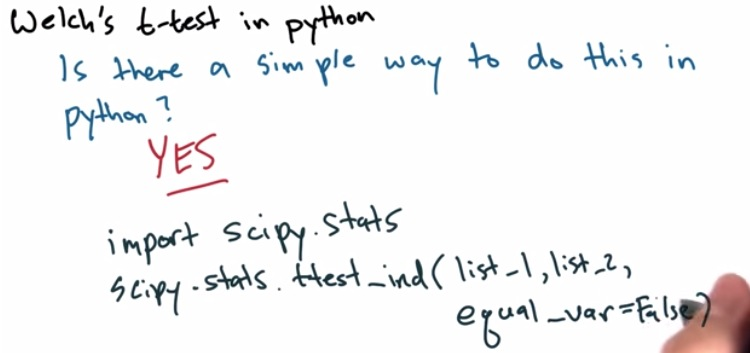• by specifyinhg equal-var=true, the calculations will be exactly the same as welch's t-test
• it will return a tuple, t and p value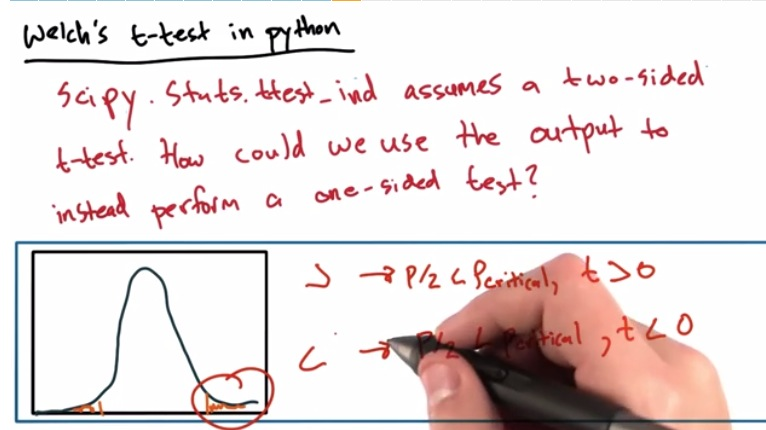• we can do it by comparing the sample with one another
• for our null hypothesis set to true, p must be less than p critical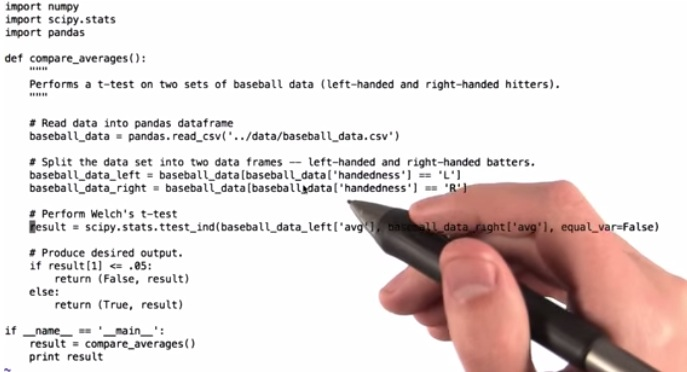• Basic implementation Welch's t-test with python